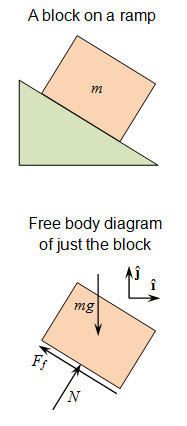## A General Approach

Basic problem-solving techniques can aid in the solution of problems involving motion (i.e., the laws of motion).

### Learning Objectives

Assess the laws of motion through practiced problem solving techniques

### Key Takeaways

#### Key Points

• Gathering all relevant information and identifying knowns and unknowns is an important first step.
• Always make a drawing to help identify directions of forces and to establish $\text{x}$, $\text{y}$, and $\text{z}$ axes.
• Choose the correct equations, solve the problem, and check that the answer fits expectations numerically.

#### Key Terms

• equation: An assertion that two expressions are equal, expressed by writing the two expressions separated by an equal sign; from which one is to determine a particular quantity.

When dealing with the laws of motion, although knowledge of concepts and equations is important, understanding basic problem solving techniques can simplify the process of solving problems that may appear difficult. Your approach to problem solving can involve several key steps.Free body diagram: An example of a drawing to help identify forces and directions.

First, gather all relevant information from the problem. Identify all quantities that are given (the knowns), then do the same for all quantities needed (the unknowns). Also, identify the physical principles involved (e.g., force, gravity, friction, etc. ).

Next, a drawing may be helpful. Sometimes a drawing can even help determine the known and unknown quantities. It need not be a work of art, but it should be clear enough to illustrate proper dimension, (meaning one, two, or three dimensions). You can then use this drawing to determine which direction is positive and which is negative (making note of this on the drawing).

A next step is to use what is known to find the appropriate equation to find what is unknown. While it is easiest to find an equation that leaves only one unknown, sometimes this is not possible. In these situations, you can solve multiple equations to find the right answer. Remember that equations represent physical principles and relationships, so use the equations and drawings in tandem.

You may then substitute the knowns into the appropriate equations and find a numerical solution.

Check the answer to see if it is reasonable and makes sense. Your judgment will improve and fine tune as you solve more problems of this nature. This “judgement” step helps intuit the problem in terms of its conceptual meaning. If you can judge whether the answer is reasonable, you have a deeper understanding of physics than simply the mechanics of problem solving.

When solving problems, we tend to perform these steps in different order, as well as do several steps simultaneously. There is no rigid procedure that will work every time. Creativity and insight grow with experience. In time, the basics of problem solving can become relatively automatic.